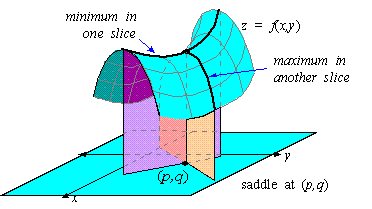Image credit: Gimages

# GAN 2

Theory of Game between Generator and Discriminator

As we saw in Intro and application of Generative Adversarial Network, GANs generate new data from a given dataset by learning the distribution of the dataset through adversarial process.

## What is an adversarial process/learning?

A google search can tell you that adversarial machine learning is a technique used in Machine Learning which tries to fool the model by giving in false/malicious input.

## Components of GANs:• ### Generator

The Generator network takes random noise as input and convert it to a data sample(image/music) . The output of generator is a fake but realistic data sample. The choice of the random noise determines the distribution into which the data sample generated falls.\
But the generator network have to be trained to produce samples for the given random noise. ie: the generator have to learn the distribution of the dataset, so it generates new data samples from the distribution.

As this is not a supervised learning, we cannot use labels to learn the parameters of generator. So we use adversarial learning technique to learn the distribution of the dataset.

The idea is to maximize the probability that the data sample generated by the generator is from the training dataset. But it is not easy as its un labelled , so we use the help of another network called Discriminator.

• ### Discriminator

Discriminator is a normal Neural Network classifier. The discriminator finds if a data sample is from the training dataset or not.\
During the training process, the discriminator is given data from the training dataset 50% of the time and data samples generated by generator other 50% of the time. The discriminator classifies the generated data samples as fake and data from the training dataset as real data.

## The Game Theory

As the disciminator classifies the data sample from the generator as fake, the generator tries to fool the discriminator by generating more realistic data sample(learns the training data distribution well). The generator starts generating samples more close to the distribution of the training dataset.As the generator tries to fool the discriminator, the discriminator learns to classify the more realistic(fake) data generated by the generator as fake. \
By this process both the networks learn the parameters which gives best results. This creates a competition between Generator(G) and Discriminator(D), this makes this an adversarial learning.

Check this

In game theory, an equlibrium is reached in a 2 player game when both the players recieve 0 payoff. When a player(P) wins, P gets a positive payoff of 1 and gets a negative payoff of -1 when loses. When a player loses, the player changes the stratergy to win the next round. As this continues the player becomes better but as the other player also gets better , an equilibrium is reached when both players uses random uniform stratergies. At equilibrium, neither of the players can improve further.\
\
Most of the machine learning models we used so far depends on optimization algorithms. We finds a set of parameters for which the cost function is minimum. But GANs have 2 players G & D. The G is trying to fool D and D is trying to classify G’s sample as fake data. As we can see D is trying to minimize the probability of G’s output as true data, whereas G is trying to increase the probability.

Cost of D = minimize(P(generated sample data is real))
Cost of G = maximize(P(generated sample data is real))


Theoritically equlibrium occurs when both probabilities are equal.

P(generated sample data is real) = 0.5


This occurs for a set of parameters for which the G got the maximum probability and D got minimum probability. ie: a saddle point.

saddle point - both local maxima and local minimaGenerator gets a local maxima when the distribution learned by generator is equal to the distribution of the training dataset.

We will use 2 seperate optimization algorithms for D and G, so it is not possible for us to find the equilibrium. But if we can use a single optimization algorithm which reduces both D & G costs together, then we may encounter perfect equilibirum.

In the next post, we will look into the practical implementation of GANs by coding and training it in PyTorch.##### Shangeth Rajaa
###### Researcher at Vernacular.ai

Machine Learning Researcher at Vernacular.ai